Prediction Trees are used to predict a response or class \(Y\) from input \(X_1, X_2, \ldots, X_n\). If it is a continuous response it’s called a regression tree, if it is categorical, it’s called a classification tree. At each node of the tree, we check the value of one the input \(X_i\) and depending of the (binary) answer we continue to the left or to the right subbranch. When we reach a leaf we will find the prediction (usually it is a simple statistic of the dataset the leaf represents, like the most common value from the available classes).

Contrary to linear or polynomial regression which are global models (the predictive formula is supposed to hold in the entire data space), trees try to partition the data space into small enough parts where we can apply a simple different model on each part. The non-leaf part of the tree is just the procedure to determine for each data \(x\) what is the model (i.e, which leaf) we will use to classify it.

One of the most comprehensible non-parametric methods is k-nearest-neighbors: find the points which are most similar to you, and do what, on average, they do. There are two big drawbacks to it: first, you’re defining “similar” entirely in terms of the inputs, not the response; second, k is constant everywhere, when some points just might have more very-similar neighbors than others. Trees get around both problems: leaves correspond to regions of the input space (a neighborhood), but one where the responses are similar, as well as the inputs being nearby; and their size can vary arbitrarily. Prediction trees are adaptive nearest-neighbor methods. - From here

## Regression Trees

Regression Trees like say linear regression, outputs an expected value given a certain output.

``````library(tree)

tree.model <- tree(log(MedianHouseValue) ~ Longitude + Latitude, data=real.estate)
plot(tree.model)
text(tree.model, cex=.75)``````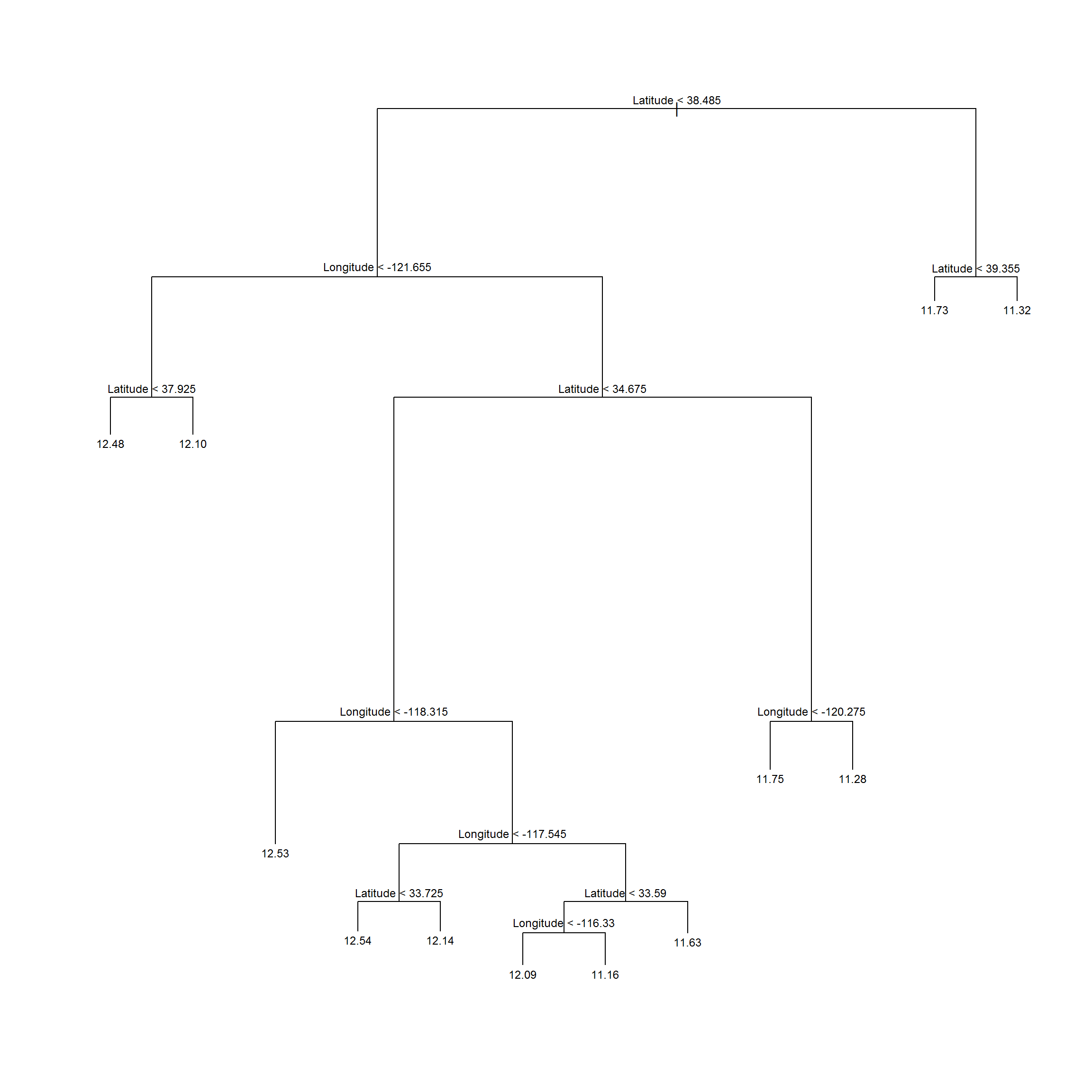Notice that the leaf values represent the log of the price, since that was the way we represented the formula in the `tree()` function.

(note: Knitr seems to output the wrong values above, check the results yourself in R)

We can compare the predictions with the dataset (darker is more expensive) which seem to capture the global price trend:

``````price.deciles <- quantile(real.estate\$MedianHouseValue, 0:10/10)
cut.prices    <- cut(real.estate\$MedianHouseValue, price.deciles, include.lowest=TRUE)
plot(real.estate\$Longitude, real.estate\$Latitude, col=grey(10:2/11)[cut.prices], pch=20, xlab="Longitude",ylab="Latitude")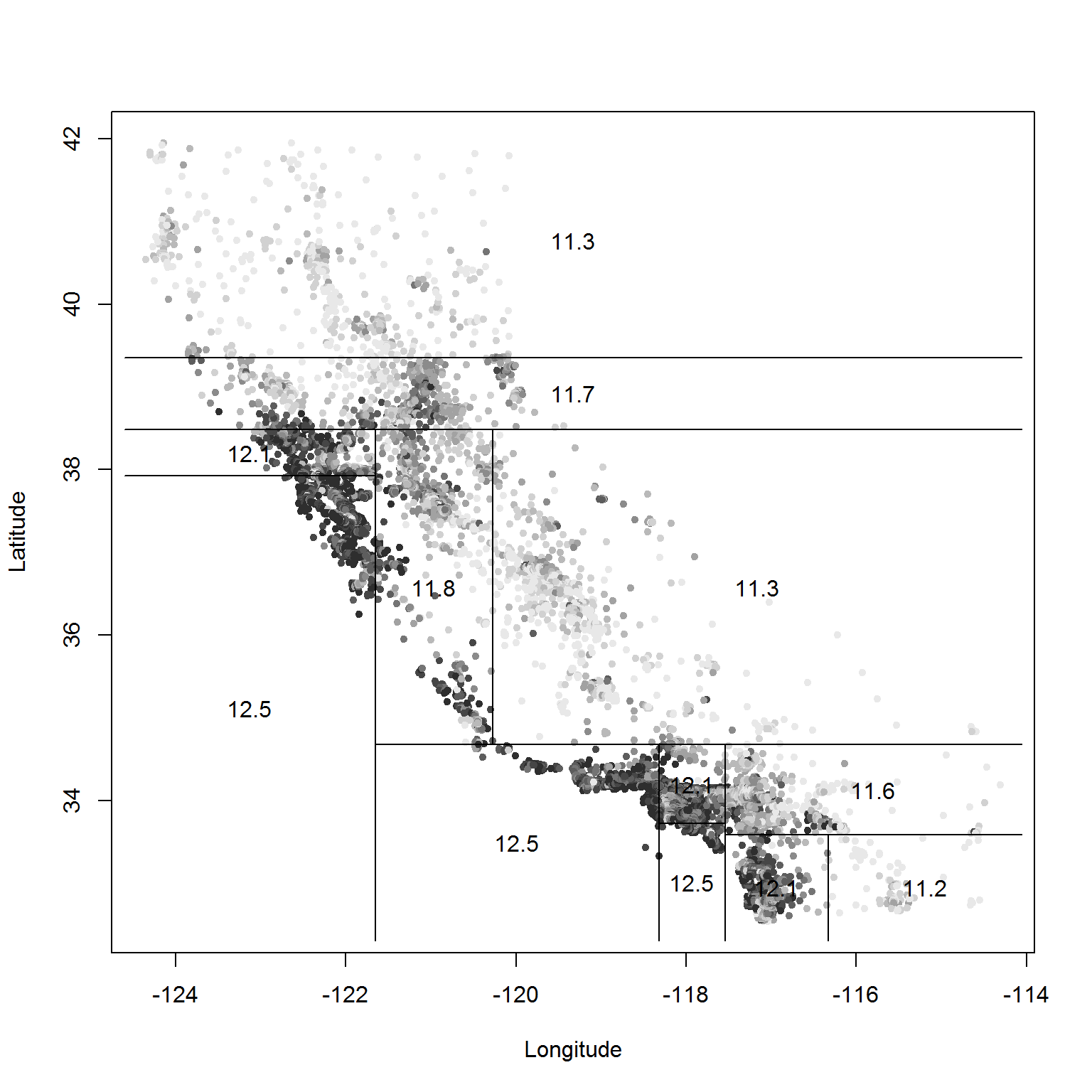``summary(tree.model)``
``````##
## Regression tree:
## tree(formula = log(MedianHouseValue) ~ Longitude + Latitude,
##     data = real.estate)
## Number of terminal nodes:  12
## Residual mean deviance:  0.1662 = 3429 / 20630
## Distribution of residuals:
##     Min.  1st Qu.   Median     Mean  3rd Qu.     Max.
## -2.75900 -0.26080 -0.01359  0.00000  0.26310  1.84100``````

Deviance means here the mean squared error.

The flexibility of a tree is basically controlled by how many leaves they have, since that’s how many cells they partition things into. The `tree` fitting function has a number of controls settings which limit how much it will grow | each node has to contain a certain number of points, and adding a node has to reduce the error by at least a certain amount. The default for the latter, `min.dev`, is 0:01; let’s turn it down and see what happens:

``````tree.model2 <- tree(log(MedianHouseValue) ~ Longitude + Latitude, data=real.estate, mindev=0.001)
plot(tree.model2)
text(tree.model2, cex=.75)``````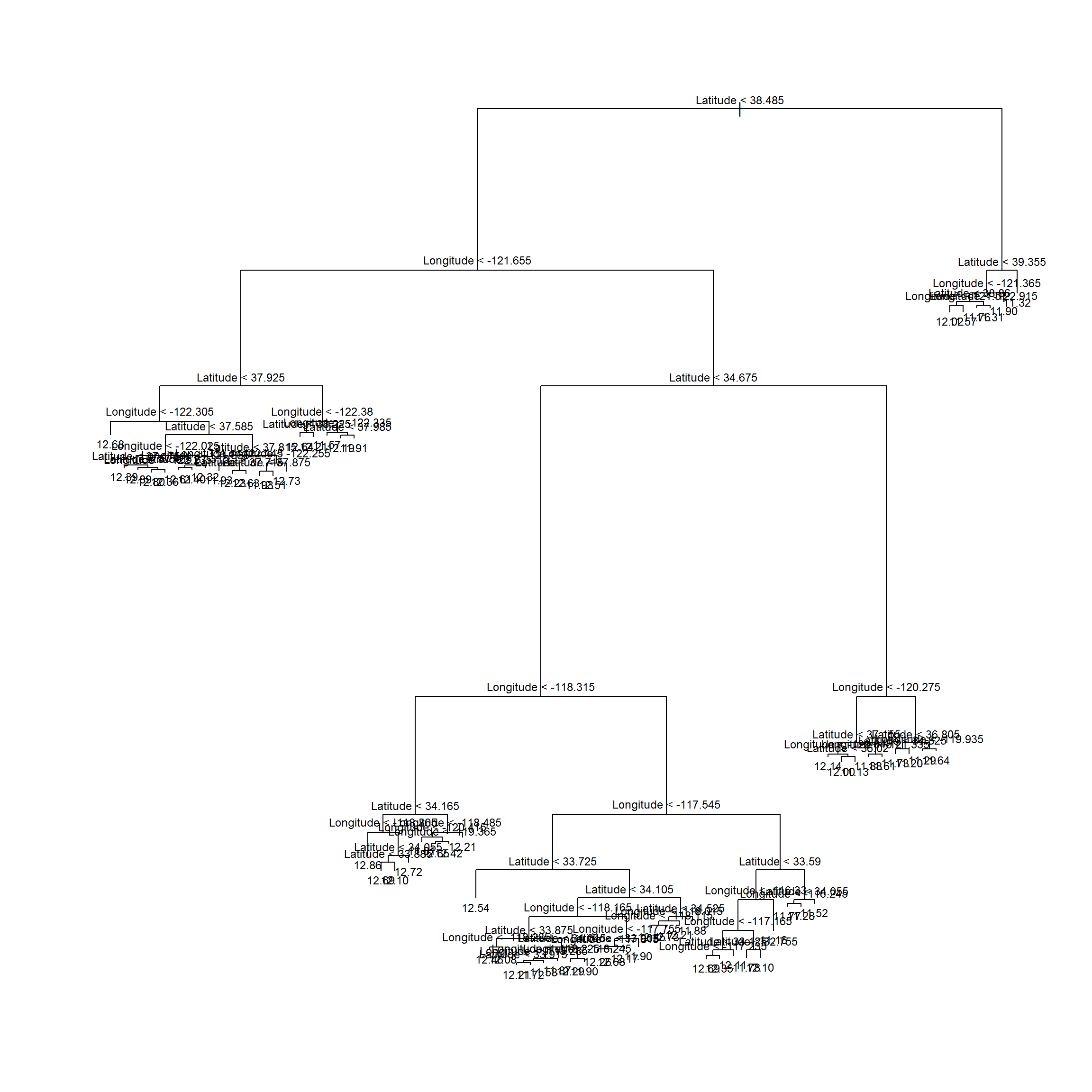``summary(tree.model2)``
``````##
## Regression tree:
## tree(formula = log(MedianHouseValue) ~ Longitude + Latitude,
##     data = real.estate, mindev = 0.001)
## Number of terminal nodes:  68
## Residual mean deviance:  0.1052 = 2164 / 20570
## Distribution of residuals:
##     Min.  1st Qu.   Median     Mean  3rd Qu.     Max.
## -2.94700 -0.19790 -0.01872  0.00000  0.19970  1.60600``````

It’s obviously much finer-grained than the previous example (68 leafs against 12), and does a better job of matching the actual prices (lower error).

Also, we can include all the variables, not only the latitude and longitude:

``````tree.model3 <- tree(log(MedianHouseValue) ~ ., data=real.estate)
plot(tree.model3)
text(tree.model3, cex=.75)``````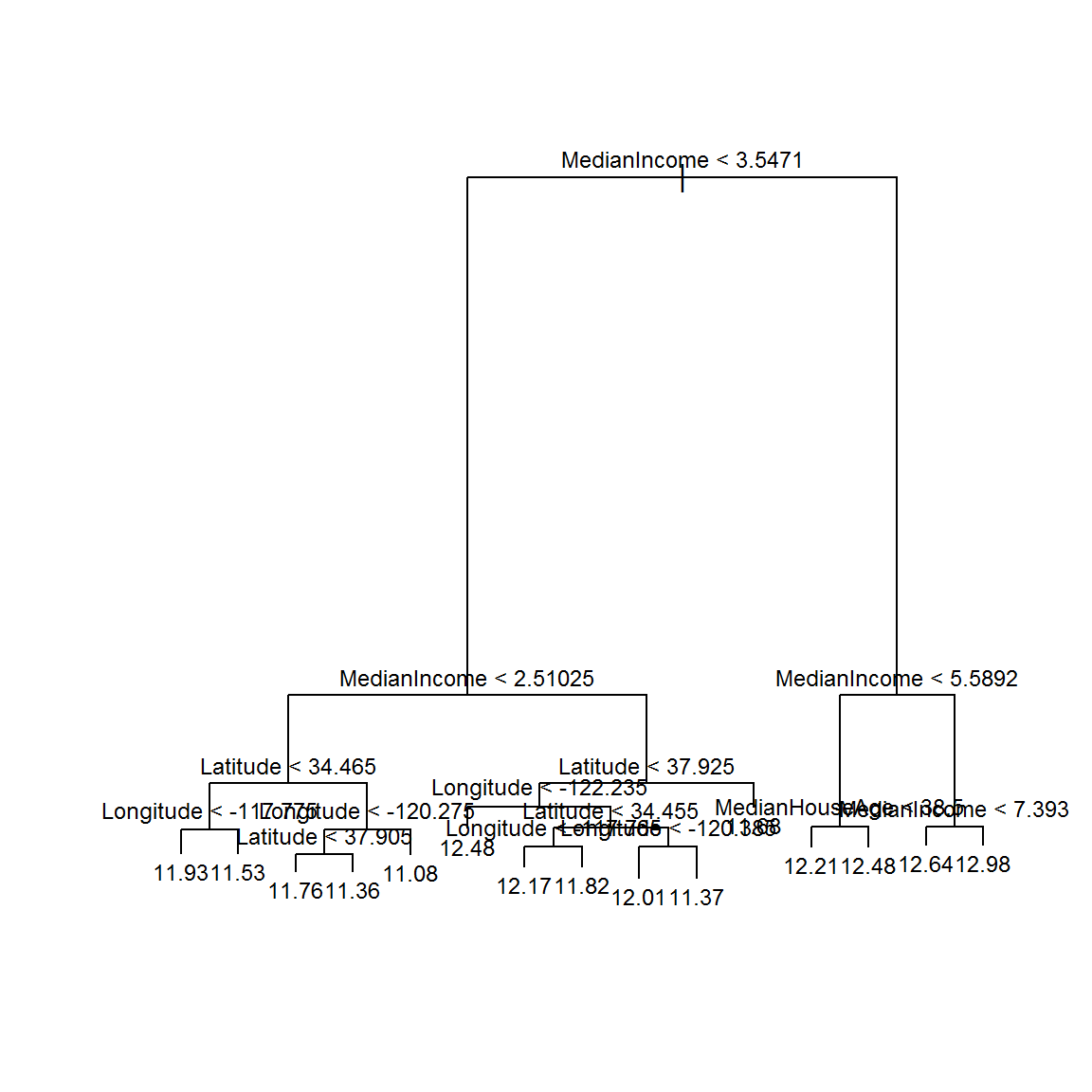``summary(tree.model3)``
``````##
## Regression tree:
## tree(formula = log(MedianHouseValue) ~ ., data = real.estate)
## Variables actually used in tree construction:
##  "MedianIncome"   "Latitude"       "Longitude"      "MedianHouseAge"
## Number of terminal nodes:  15
## Residual mean deviance:  0.1321 = 2724 / 20620
## Distribution of residuals:
##     Min.  1st Qu.   Median     Mean  3rd Qu.     Max.
## -2.86000 -0.22650 -0.01475  0.00000  0.20740  2.03900``````

## Classification Trees

Classification trees output the predicted class for a given sample.

Let’s use here the iris dataset (and split it into train and test sets):

``````set.seed(101)
alpha     <- 0.7 # percentage of training set
inTrain   <- sample(1:nrow(iris), alpha * nrow(iris))
train.set <- iris[inTrain,]
test.set  <- iris[-inTrain,]``````

There are two options for the output: + Point prediction: simply gives the predicted class + Distributional prediction: gives a probability for each class

``````library(tree)

tree.model <- tree(Species ~ Sepal.Width + Petal.Width, data=train.set)
tree.model``````
``````## node), split, n, deviance, yval, (yprob)
##       * denotes terminal node
##
##  1) root 105 230.200 versicolor ( 0.33333 0.36190 0.30476 )
##    2) Petal.Width < 0.8 35   0.000 setosa ( 1.00000 0.00000 0.00000 ) *
##    3) Petal.Width > 0.8 70  96.530 versicolor ( 0.00000 0.54286 0.45714 )
##      6) Petal.Width < 1.7 40  21.310 versicolor ( 0.00000 0.92500 0.07500 )
##       12) Petal.Width < 1.35 20   0.000 versicolor ( 0.00000 1.00000 0.00000 ) *
##       13) Petal.Width > 1.35 20  16.910 versicolor ( 0.00000 0.85000 0.15000 )
##         26) Sepal.Width < 3.05 14  14.550 versicolor ( 0.00000 0.78571 0.21429 ) *
##         27) Sepal.Width > 3.05 6   0.000 versicolor ( 0.00000 1.00000 0.00000 ) *
##      7) Petal.Width > 1.7 30   8.769 virginica ( 0.00000 0.03333 0.96667 )
##       14) Petal.Width < 1.85 8   6.028 virginica ( 0.00000 0.12500 0.87500 ) *
##       15) Petal.Width > 1.85 22   0.000 virginica ( 0.00000 0.00000 1.00000 ) *``````
``summary(tree.model)``
``````##
## Classification tree:
## tree(formula = Species ~ Sepal.Width + Petal.Width, data = train.set)
## Number of terminal nodes:  6
## Residual mean deviance:  0.2078 = 20.58 / 99
## Misclassification error rate: 0.0381 = 4 / 105``````
``````# Distributional prediction
my.prediction <- predict(tree.model, test.set) # gives the probability for each class
``````##    setosa versicolor virginica
## 5       1          0         0
## 10      1          0         0
## 12      1          0         0
## 15      1          0         0
## 16      1          0         0
## 18      1          0         0``````
``````# Point prediction
# Let's translate the probability output to categorical output
maxidx <- function(arr) {
return(which(arr == max(arr)))
}
idx <- apply(my.prediction, c(1), maxidx)
prediction <- c('setosa', 'versicolor', 'virginica')[idx]
table(prediction, test.set\$Species)``````
``````##
## prediction   setosa versicolor virginica
##   setosa         15          0         0
##   versicolor      0         11         1
##   virginica       0          1        17``````
``````plot(tree.model)
text(tree.model)``````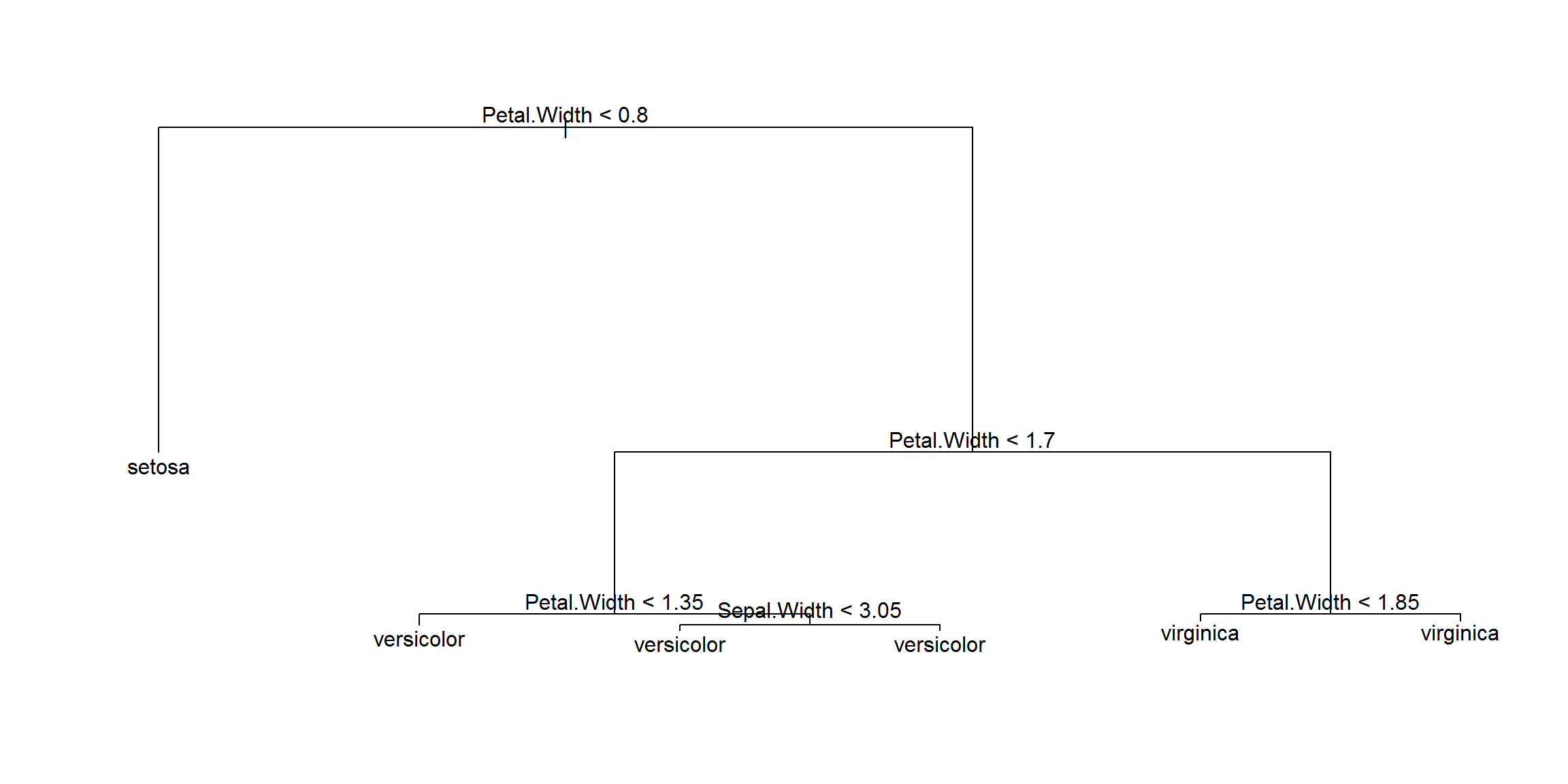``````# Another way to show the data:
plot(iris\$Petal.Width, iris\$Sepal.Width, pch=19, col=as.numeric(iris\$Species))
legend("topright",legend=unique(iris\$Species), col=unique(as.numeric(iris\$Species)), pch=19)``````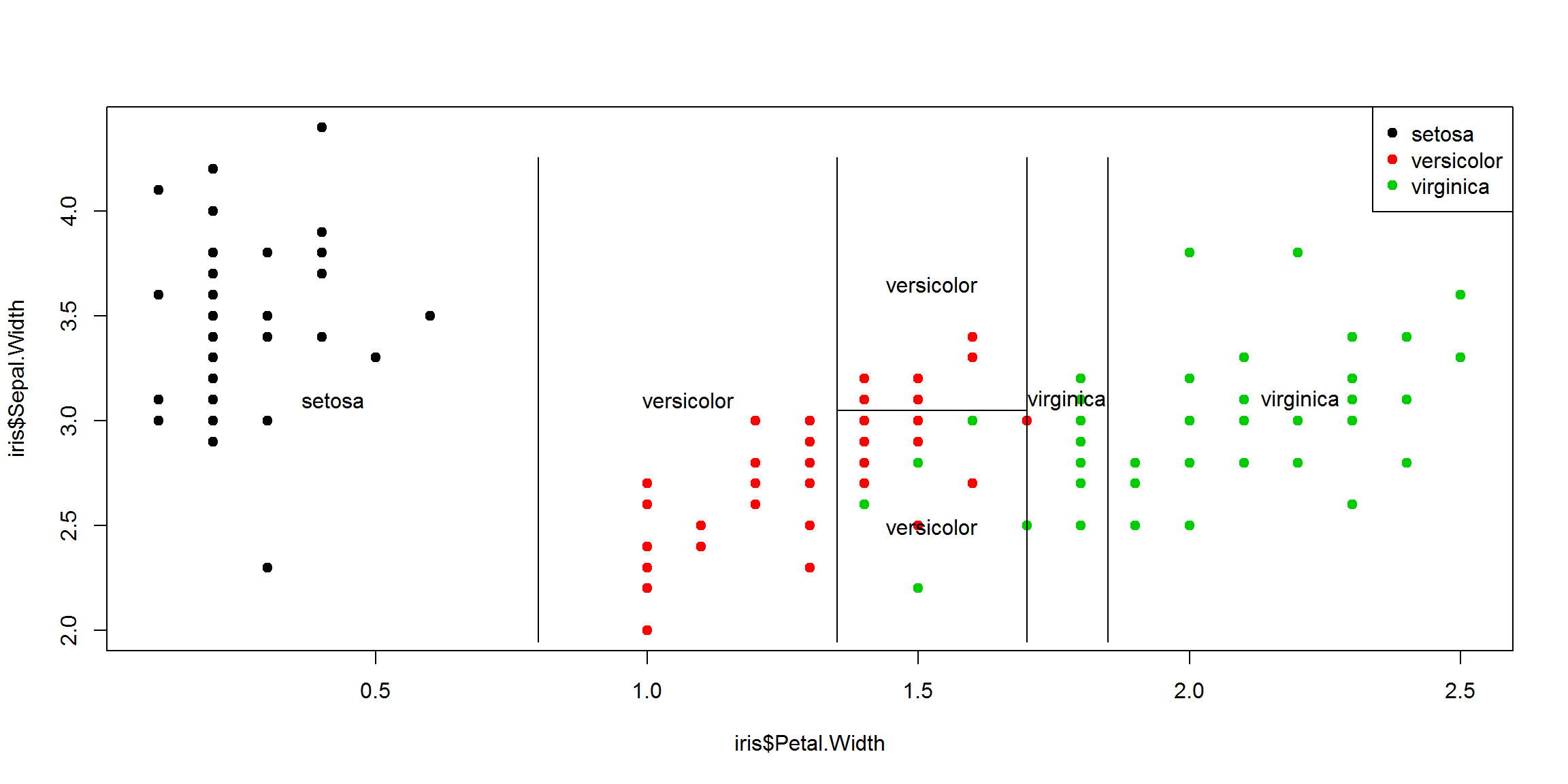``summary(tree.model)``
``````##
## Classification tree:
## tree(formula = Species ~ Sepal.Width + Petal.Width, data = train.set)
## Number of terminal nodes:  6
## Residual mean deviance:  0.2078 = 20.58 / 99
## Misclassification error rate: 0.0381 = 4 / 105``````

We can prune the tree to prevent overfitting. The next function `prune.tree()` allows us to choose how many leafs we want the tree to have, and it returns the best tree with that size.

The argument `newdata` accepts new input for making the prune decision. If new data is not given, the method uses the original dataset from which the tree model was built.

For classification trees we can also use argument `method="misclass"` so that the pruning measure should be the number of misclassifications.

``````pruned.tree <- prune.tree(tree.model, best=4)
plot(pruned.tree)
text(pruned.tree)``````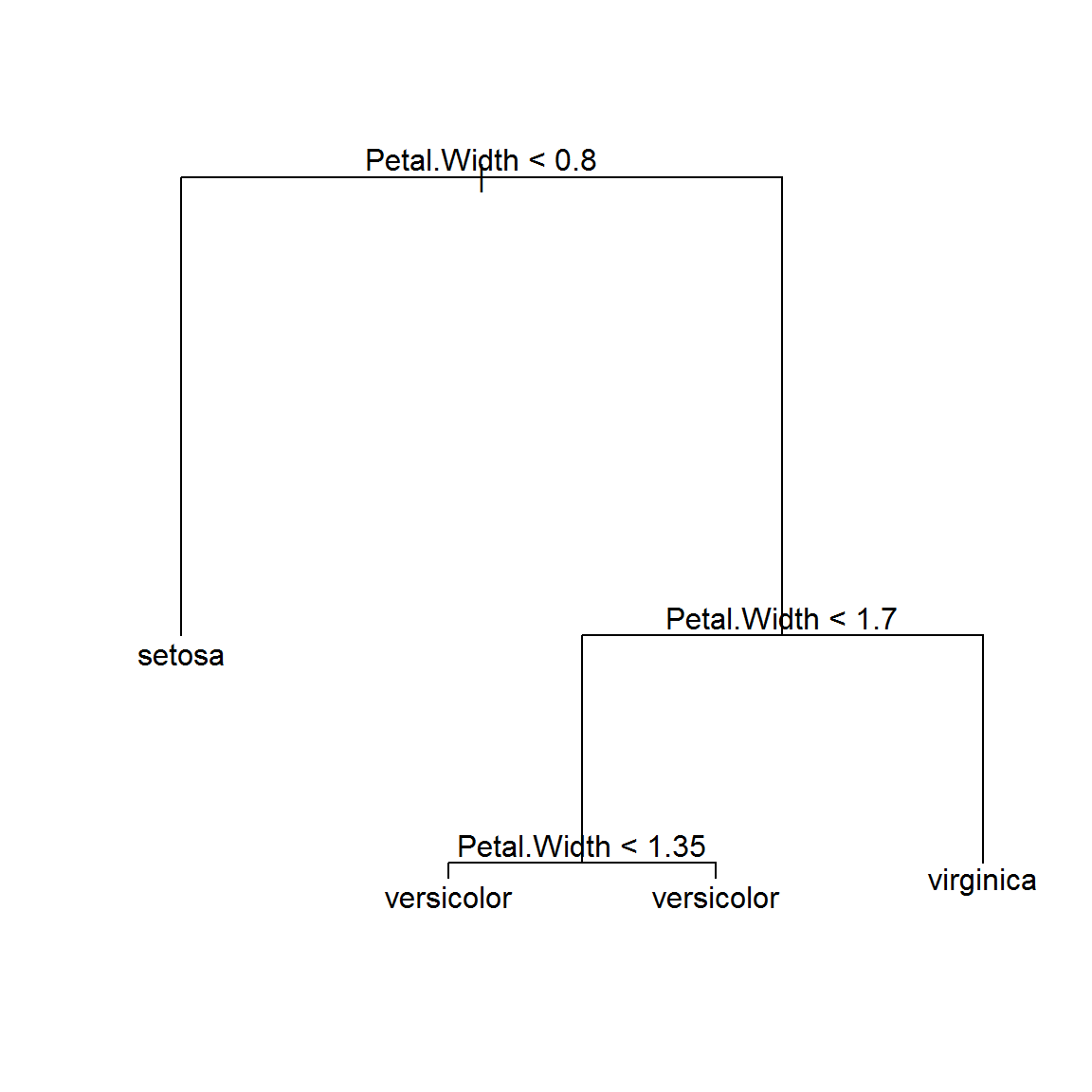``````pruned.prediction <- predict(pruned.tree, test.set, type="class") # give the predicted class
table(pruned.prediction, test.set\$Species)``````
``````##
## pruned.prediction setosa versicolor virginica
##        setosa         15          0         0
##        versicolor      0         11         1
##        virginica       0          1        17``````

This package can also do K-fold cross-validation using `cv.tree()` to find the best tree:

``````# here, let's use all the variables and all the samples
tree.model <- tree(Species ~ ., data=iris)
summary(tree.model)``````
``````##
## Classification tree:
## tree(formula = Species ~ ., data = iris)
## Variables actually used in tree construction:
##  "Petal.Length" "Petal.Width"  "Sepal.Length"
## Number of terminal nodes:  6
## Residual mean deviance:  0.1253 = 18.05 / 144
## Misclassification error rate: 0.02667 = 4 / 150``````
``````cv.model <- cv.tree(tree.model)
plot(cv.model)``````